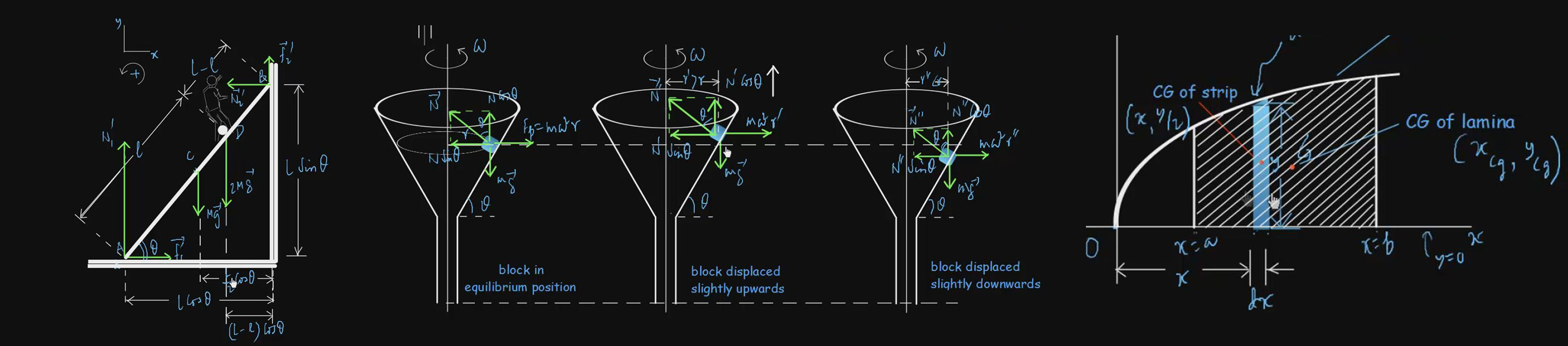# Friction

We often simplify problems by assuming that the motion of bodies takes place on “frictionless” surfaces. Strictly speaking, there is no such surface. In real life all motions happening around us are affected by the force of friction. Therefore, a realistic approach to any mechanical problem requires that we identify the frictional forces acting on the system and include them in the respective equations of motion. That is precisely what you will learn to do in the present topic. You will find video lectures on Static and Kinetic friction, Angle of Repose, Angle of Friction, Examples of Motion on Rough Surfaces, Rolling Friction, Drag Force etc. explained and supported by suitable applications and numerical examples.

#### Basic level videos

Angle Of Repose And Angle Of Friction 1:06:42 Basic
350 7.5
Drag Force 50:02 Basic
300 5
Examples Of Motion On Rough Surfaces I & II 49:14 Basic
250 4
Examples Of Motion On Rough Surfaces III 1:01:19 Basic
350 5
Problems On Drag Force 31:27 Basic
250 4
Problems On Motion On Rough Surfaces 52:27 Basic
300 5
Problems On Motion On Rough Surfaces II 1:09:32 Basic
350 7.5
Problems On Motion On Rough Surfaces III 45:08 Basic
250 4
Rolling Friction 1:01:54 Basic
350 7.5
Static And Kinetic Friction 1:02:11 Basic
350 7.5
Examples Of Motion On Rough Surfaces IV 1:11:34 Basic
350 7.5

#### Advanced level Videos Note: (CE) Stands for Problems from Competitive Examination Papers

Advanced-Level Problems On Friction I 54:56
300
5
Advanced-Level Problems On Friction II 1:15:46
350
7.5